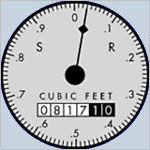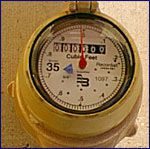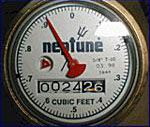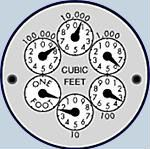## How to Read the MeterYour water meter is generally located near the curb in front of your home although in some areas (usually cold climates) it may be inside your home usually in the basement. Outside meters are typically housed in a concrete box usually marked "water" (as shown in the photo) or in a meter pit with a cast iron lid. Carefully remove the lid by using a tool such as a large screwdriver or pliers. Visually examine the area around the meter to make sure there are no harmful insects or other animals.

Water meters in the U.S. typically measure volume in gallons or cubic feet. One cubic foot = 7.48 gallons and 100 cubic feet = 748 gallons. Water charges are typically based on 100 cubic feet or on 1000 gallon units.

## Water Meter Types

There are two basic types of water meters -- the straight-reading meter which resembles an odometer in a car, and the round-reading meter which has several separate dials. The "straight-reading" meter is by far the most common.

Figure 1In the meter shown in Figure 1, the reading is taken from the figures shown under the words CUBIC FEET. The meter reads 81710.03 which is the total number of cubic feet of water recorded since the meter was installed. If the utility bills in units of 100 cubic feet they would read this meter as simply 817.

Figure 2The meter shown in Figure 2 is brand new; hence, the reading for this meter is 0.00. The small blue triangle (just to the right of the "35") is the low flow indicator. That triangle will spin if any water is flowing through the meter. This indicator can be useful in leak detection.

Figure 3The meter in Figure 3, also cubic feet, is good example of a situation where the final number has already "turned over". The correct reading on this meter is: 2425.92 cubic feet. On most meters, the final digit will turn over once the big sweep hand has passed the 0.6 mark. Note that the size of the meter is usually printed on the dial. The meter in Figure 4 is a 5/8" meter as is shown on the dial.

Figure 4The meter in Figure 4 is an older style and is much less common; however, there are still some of these meters in service. This type of meter has several small dials and is a little more difficult to read than the straight-reading meter. The dials are marked off in divisions of 10, and are read much like a clock, except that the hand on every other dial turns counterclockwise. To read this meter, begin with the 100,000 dial and read each dial around the meter to the one foot dial. If the hand is between numbers, use the lower number. Therefore, the dials at right register 806323.